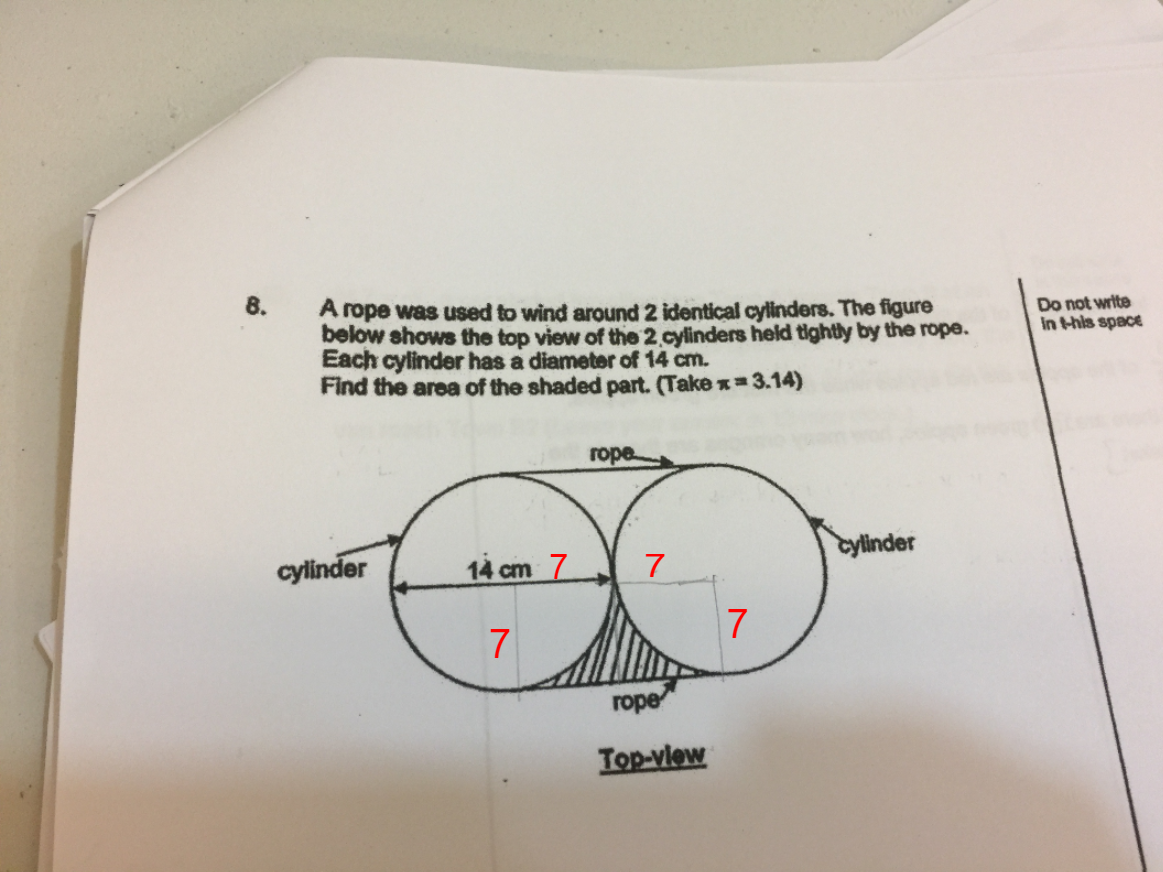# QuestionSince you already drawn the small rectangle in the diagram, we will use that.

Area of rectangle = 14 X 7 = 98 cm2

Area of the 2 quadrant in the rectangle = Pi X 7 X7 /2 = 76.93 cm2

Area of shaded portion = 98 – 76.93 =  21.07 cm2 ##